You've successfully subscribed to ムえのBLOG
Great! Next, complete checkout for full access to ムえのBLOG
Welcome back! You've successfully signed in.
Success! Your account is fully activated, you now have access to all content.. 3 min read

# Lecture 5 RC/RL First-Order Circuits

## Motivation

Previously, we only have static analysis of a circuit.

Two assumptions:

1. Responses at time t only depend on inputs at time t.
2. Circuits respond to input change infinitely fast.

What if without this two assumptions?

dynamic circuit analysis

## Capacitors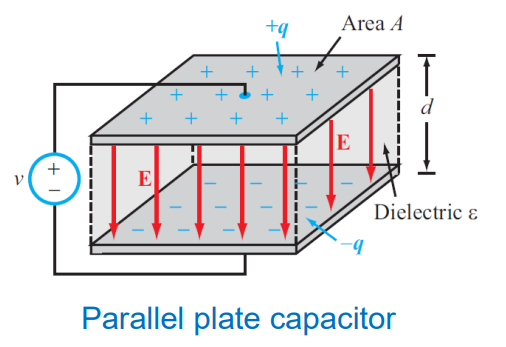1. Passive element
2. Storage element that stores energy in electric field

$$\epsilon 为中间绝缘电介质的介电常数$$
$$E为电场$$
$$C = \frac{\epsilon S}{4\pi kd}$$
$$Q = C V$$

How does current flow through a capacitor?

​ Through charging and discharging the two plates, it seems like current flow through a capacitor.

### V-I characteristic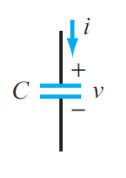$$q(t_0) = CV(t_0)$$
$$q(t) = CV(t)$$
$$\int_{t_0}^{t} i(\tau) d\tau = q(t)-q(t_0)$$
$$V(t) = \frac{1}{C}\int_{t_0}^ti(\tau)d\tau +V(t_0)$$
$$i(t) = C \frac{dV(t)}{dt}$$

### Stored Energy

$$P(t) = V(t)i(t) = V(t)C\frac{dV(t)}{dt}$$
$$E = \int_{t_0}^t p(\tau)d\tau =\int_{t_0}^t V(\tau)C \frac{dV(\tau)}{d\tau}d\tau$$
$$=\frac{1}{2}C[V^2(t)-V^2(t_0)]$$

The energy stored in a capacitor with voltage $V(t)$ is
$$W = E(start , from , V(t_0)=0) = \frac{1}{2}CV^2(t)$$

### Important Property

$$i(t) = C \frac{dV(t)}{dt}$$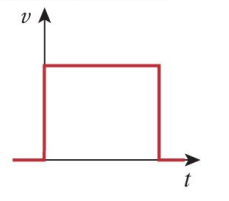Is it possible?

If at $t=0$ then $i(t)\arrowvert_{ t = 0 }=\infty$ so it is impossible.

#### Property 1

$V(t)$ cannot change instantaneously

#### Property 2

$V(t):DC \Rightarrow \frac{dV(t)}{dt}=0 \Rightarrow i(t)=0 \Rightarrow opencircuit$

### Capacitors in Series or in Parallel 串并联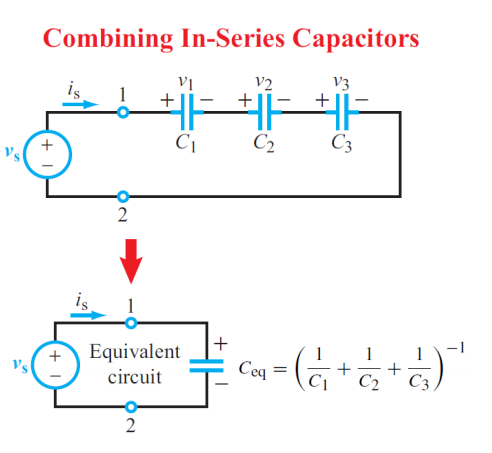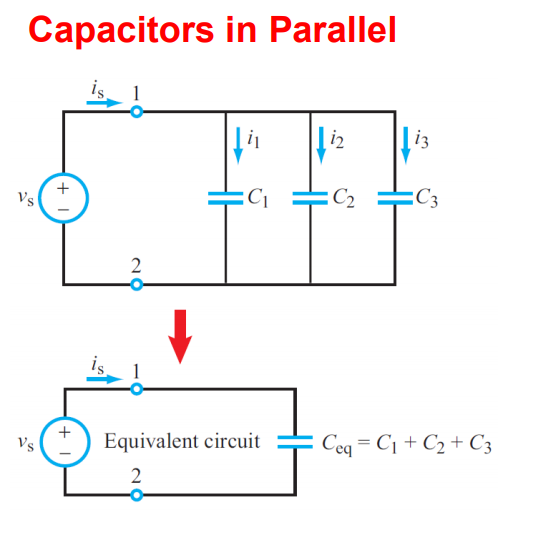#### voltage division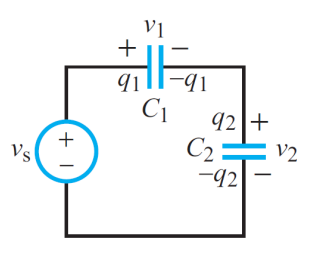$$v_1 = (\frac{C_2}{C_1+C_2})v_s$$
$$v_2 = (\frac{C_1}{C_1+C_2})v_s$$

#### current division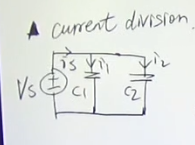$$i_1 = (\frac{C_1}{C_1+C_2})i_s$$
$$i_2 = (\frac{C_2}{C_1+C_2})i_s$$

### practical capacitors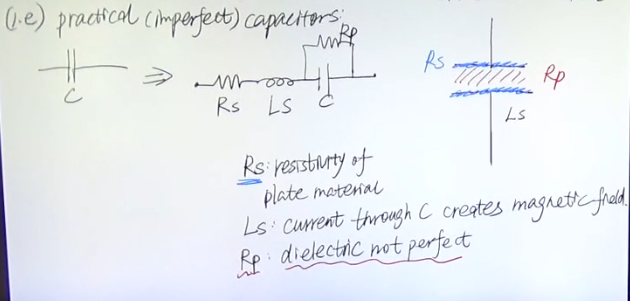## Inductors

1. Passive element

2. A storage element that stores energy in magnetic field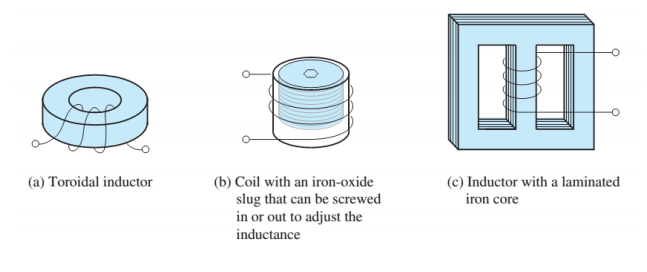$$L = \frac{N^2\mu S}{l}$$
$$\Lambda=L\cdot i=N\cdot B\cdot S=N\phi$$

$N$匝数，$\Lambda$ 磁通链

### V-I characteristic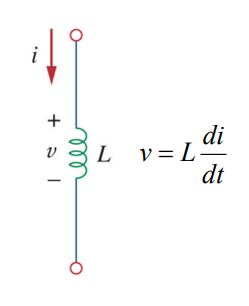$$V(t)=\frac{d\Lambda(t)}{dt}=\frac{d(li(t))}{dt}=L\frac{di(t)}{dt} \$$